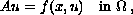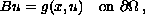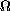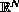Electron. J. Diff. Eqns., Vol. 1998(1998), No. 10, pp. 1-13.

### Existence and multiplicity of solutions to a p-Laplacian equation with nonlinear boundary condition Klaus Pflueger

Abstract:
We study the nonlinear elliptic boundary value problemwhere A is an operator of p-Laplacian type,is an unbounded domain inwith non-compact boundary, and f and g are subcritical nonlinearities. We show existence of a nontrivial nonnegative weak solution when both f and g are superlinear. Also we show existence of at least two nonnegative solutions when one of the two functions f, g is sublinear and the other one superlinear. The proofs are based on variational methods applied to weighted function spaces.

Submitted March 5, 1998. Published April 10, 1998.
Math Subject Classification: 35J65, 35J20.
Key Words: p-Laplacian, nonlinear boundary condition, variational methods, unbounded domain, weighted function space.

Show me the PDF file (163 KB), TEX file, and other files for this article.Klaus Pflueger FB Mathematik, Freie Universitat Berlin Arnimallee 3, 14195 Berlin, Germany e-mail: pflueger@math.fu-berlin.de Web page: http://www.math.fu-berlin.de/user/pflueger